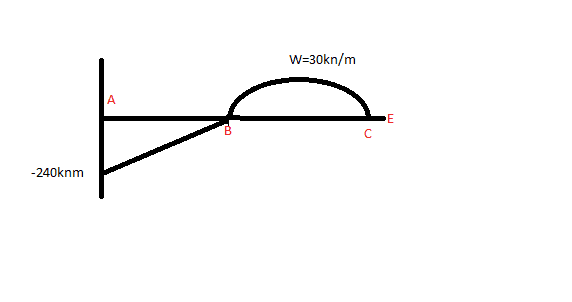# Bending moment diagrams with a hinge?

## Homework Statement

Hey guys I'm having trouble drawing the bending moment diagram for this beam with a hinge?
I'm not sure where to start? I've looked at stuff online and its completely different to the stuff in my textbook

## Homework Equations

equilibrium equations
ƩFx=0 ƩFy=0 ƩM=0
Am I right to say that Ay=0 because the moment at the hinge is 0?
ƩMb=0 so
4m*Ay=0 --> Ay=0?

## The Attempt at a Solution

I know you start with the equilibrium equations to find the reactions but I'm not sure that what I am doing is right?
Any help with drawing the BMD would be appreciated :)

## The Attempt at a Solution

#### Attachments

•photo.jpg
51.9 KB · Views: 3,398

## Answers and Replies

The hinge is the key to the problem because the bending moment there is zero. You can take moments to the right of the hinge, or to the left. AY is not zero because you have ignored the moment at A. Have you drawn a free body diagram and put on it all the actions and reaction components?

Solving the forces gives
Ay=30kN
Cy=90kN
If I take the moment at B
4Ay+Ma=0
Ma=-120kNm??

There is a mistake in your latest post. You need to show your working. Taking moments about B to the right of B, what equation do you get? Can I ask you to state what definition of bending moment you are using (there are two possible)?

To the right of B
0=(30*4*2)-4Cy
Which means Cy=60kN??
Umm On the left side counterclock wise is positive and on the right clockwise is positive?

Yes Cy=60 upwards. Sign conventions vary. Just stick to one while you are learning. The sign is not related to clockwise or anticlockwise but relates to whether the beam is hogging (convex upwards) or sagging(convex downwards) from the point of view of an observer under the beam. Now you have Cy, you can get the reaction components at A because there are 3 unknowns and 3 equations. These statically determinate problems are all self-checking. You don't need to ask if you have got it right. For example, in this case, when you have got all the reaction components, you should be able to check vertical equilibrium and take moments about E for the whole thing to check the moments are zero there. I hope you are successful, because it's a good learning problem.

i calculated my reaction forces the vertical reaction at point a equal to 60kn and vertical reaction point at c is also 60kn
trying to calculate the bending moment at point A , i said sum of the moment at A equal to zero and my positive moment is clockwise. my equation is M(a)+(30*4*6)-(60*8)=0 so moment at A equal to -240knm. what confusing is how this beam is deflecting. do i take into account of the moment at point C when i am drawing my bending moment diagram?

well done. deflection: AB is hogging. Imagine a cut off beam as a cantilever AB with a point load of 60 on its end at B. It will deflect down and cause a moment of -240 at A just like you have. So B moves down and AB is hogging. The hinge at B shows a change of gradient there. Then BC is sagging because of the load in it. What about CE? There is no load on it. So it remains straight but inclined witht he same gradient as found just to the left of C. You didn't answer my question about the definition of Bending moment at a section. But if you did answer it, you would answer also your question "do i take into account of the moment at point C when i am drawing my bending moment diagram?"

will have a bending moment digram ofYour bending moment diagram is actually quite promising and is correct in the two end sections, but there are three errors. The first is that the gradient just to the left of B should be the same as the gradient just to the right of B. Not quite. The slope in BA is an asymptote to the diagram in BC. In BC. Your diagram in BC looks elliptical, perhaps restrained by the drawing program you are using. It should be a parabola with the 'sharpest point' at the top. If you extend the diagram's straight line in AB to meet the perpendicular bisector of BC, you will get a value twice the moment at that point. That gives you a correct scale to the parabolic part of the diagram. If this were an exam I would pass it with 56%.